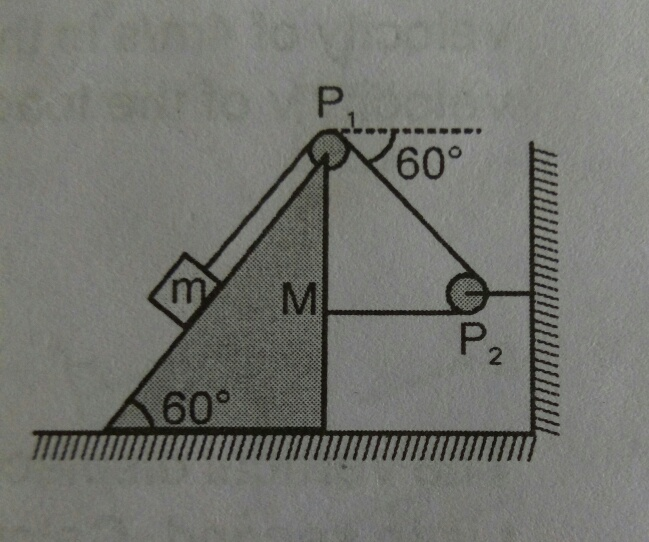# Annoying WedgeIn the arrangement shown above, the block of mass $m=2 \text{ kg}$ lies on the wedge of mass $M=8\text{ kg}$. Find the initial acceleration of the wedge if the surfaces are smooth, pulley and strings are massless.

If the magnitude of acceleration is in the form $\dfrac{a\sqrt{b}}{c}$, where $a,b$ and $c$ are positive integers, with $a,c$ coprime and $b$ square-free, submit your answer as $a+b+c$.

×